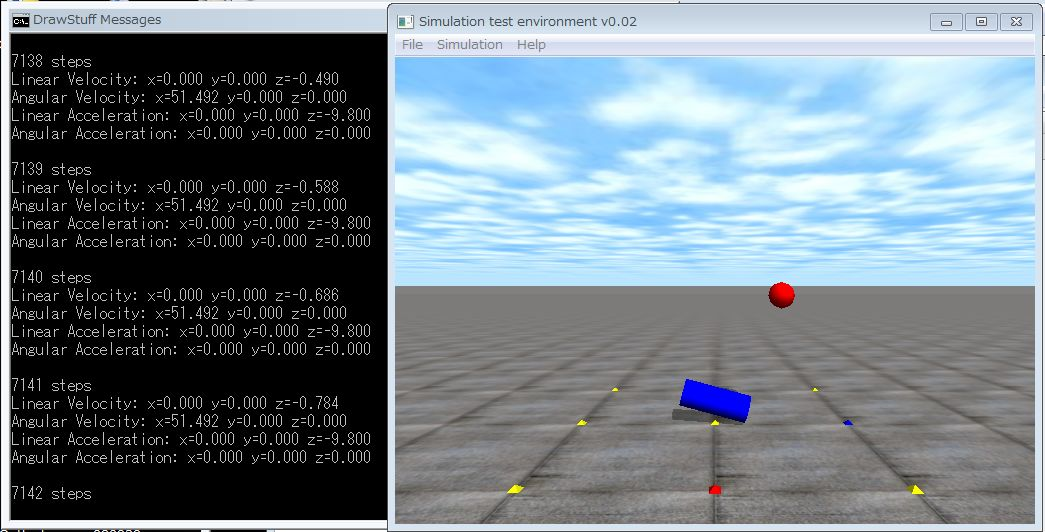# 10. Velocity and AccelerationThis tutorial explains speed, angular velocity and acceleration and angular acceleration.

1.Velocity and angular velocity

dBodySetLinearVel() sets a linear velocity, and dBodySetAngularVel()sets an angular velocity of a body. The velocity is the speed of the center of gravity and angular velocity is around the center of gravity. You may set velocity and angular velocity in the initial state.  Setting those in every simulation step is not recommended.  It abuses your physics model.

If you want to control the speed of an object. Please use a joint motor.

• void dBodySetLinearVel (dBodyID body, dReal x, dReal y, dReal z);
Set a linear velocity (x, y, z) [m/s] of a body.
• void dBodySetAngularVel (dBodyID body, dReal x, dReal y, dReal z);
Set an angular velocity (x, y, z) [rad/s].
• const dReal * dBodyGetLinearVel (dBodyID body);
Get a linear velocity (x, y, z) [m/s] of a body. The return value is a pointer to an array.
• const dReal * dBodyGetAngularVel (dBodyID body);
Get an angular velocity (x, y, z) [rad/s] of a body. The return value is a pointer to an array.

2. Acceleration and angular acceleration

Unfortunately, there are no APIs to get acceleration and angular acceleration. If you want them. divide the change of velocity by the step size, and the time step is the second argument of dWorldStep(dWorldID, dReal stepsize) . The stepsize is a time step size which is used in the numerical integration.

As a point of attention, dBodyGetLinearVel (), dBodyGetAngularVel () returns a pointer to an array composed of three elements (x, y, z).

3. Sample program

This sample program is based on the sample program 9. Only adding display about the speed, acceleration of objects.

```static void simLoop (int pause)
{
static long steps = 0;
const dReal stepsize = 0.01;
const dReal *linear_vel, *angular_vel;
static dReal linear_vel_old, angular_vel_old;
dReal linear_accel, angular_accel;

if (!pause)     {
dSpaceCollide(space,0,&nearCallback);
dWorldStep(world,stepsize);
dJointGroupEmpty(contactgroup);
}      /

// Linear velocity, Angular Velocity
linear_vel  = dBodyGetLinearVel(ball.body);
angular_vel = dBodyGetAngularVel(pillar.body);
printf("\n%d steps \n", steps++);
printf("Linear Velocity: x=%.3f y=%.3f z=%.3f \n",
linear_vel,linear_vel,linear_vel);
printf("Angular Velocity: x=%.3f y=%.3f z=%.3f \n",       angular_vel,angular_vel,angular_vel);

// Linear acceleration, Angular acceleration
for (int i=0; i < 3; i++) {
linear_accel[i] = (linear_vel[i]-linear_vel_old[i])  /stepsize;
angular_accel[i] = (angular_vel[i]-angular_vel_old[i])/stepsize;
}

printf("Linear Acceleration: x=%.3f y=%.3f z=%.3f \n",       linear_accel,linear_accel,linear_accel);
printf("Angular Acceleration: x=%.3f y=%.3f z=%.3f \n",       angular_accel,angular_accel,angular_accel);

for (int j=0; j < 3; j++) {
linear_vel_old[j]  = linear_vel[j];
angular_vel_old[j] = angular_vel[j];
}
drawObject(ball.geom,1.3,0,0);
drawObject(pillar.geom,0,0,1.3);
}```

That’s all. See you !

demu

スポンサーリンク
demura.netをフォローするdemura.net

### Folding@home Kanazawa (ID 257261)

みんなのためにおうちで新型コロナウイルスを解析しよう！

タイトルとURLをコピーしました